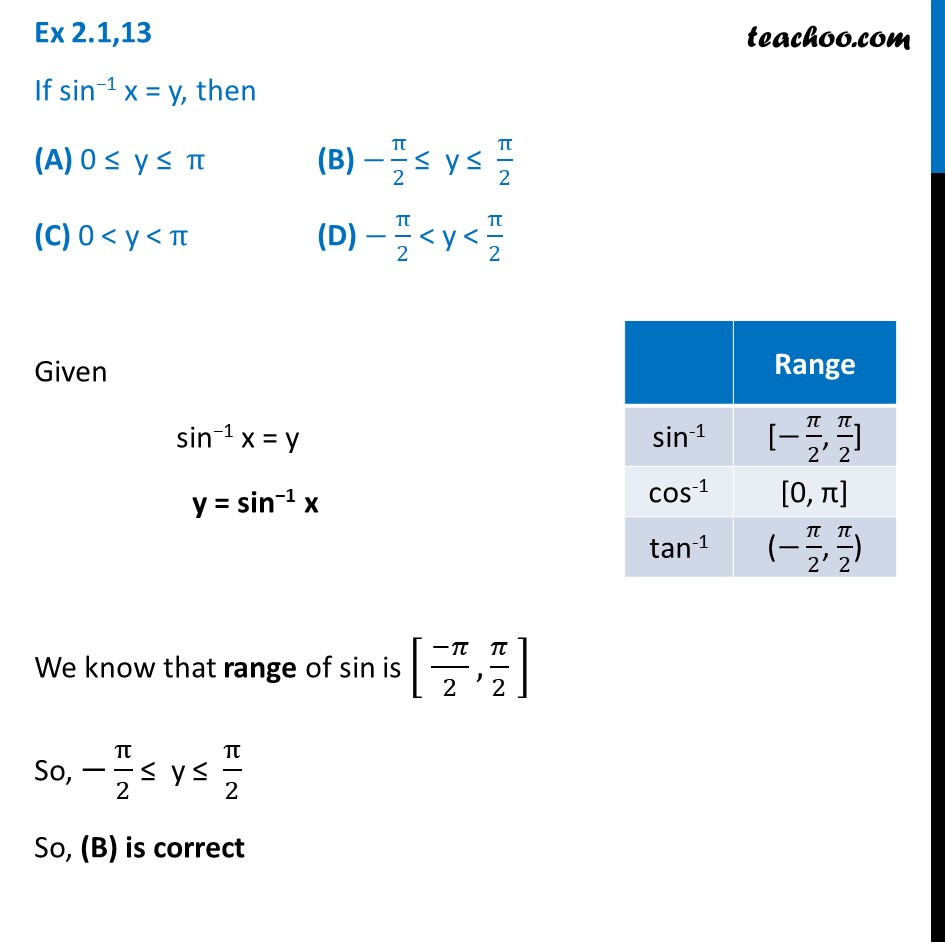1. Chapter 2 Class 12 Inverse Trigonometric Functions (Term 1)
2. Concept wise
3. Definition

Transcript

Ex 2.1,13 If sin−1 x = y, then (A) 0 ≤ y ≤ π (B) −π/2 ≤ y ≤ π/2 (C) 0 < y < π (D) − π/2 < y < π/2 Given sin−1 x = y y = sin−1 x We know that range of sin is [ (−𝜋)/2, 𝜋/2 ] So, −π/2 ≤ y ≤ π/2 So, (B) is correct